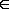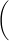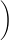Let C(n) be the constant term in the expansion of

(x + 4)n.

Prove by induction that

C(n) = 4n

for all nN.

(Induction on n.) The constant term of

(x + 4)1

is

= 4.

Suppose as inductive hypothesis that the constant term of

(x + 4)k − 1

is

for some

k > 1.

Then (x + 4)k = (x + 4)k − 1 ·, so its constant term is

· 4 =

, as required.

C(1)=4, so base case satsfies C(n)=4ⁿ when n=1.

Assume C(n)=4ⁿ. C(n+1)=(x+4)C(n)=xC(n)+4C(n)=xC(n)+4×4ⁿ. The constant in this expression is 4×4ⁿ=4ⁿ⁺¹.

But 4ⁿ⁺¹=C(n+1), which proves by induction that C(n)=4ⁿ for n∊ℕ.

by Top Rated User (775k points)